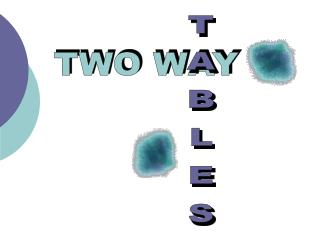DownloadDownload PresentationTWO WAY

# TWO WAY

Download Presentation## TWO WAY

- - - - - - - - - - - - - - - - - - - - - - - - - - - E N D - - - - - - - - - - - - - - - - - - - - - - - - - - -
##### Presentation Transcript

1. TWO WAY TABLES

2. Task One – Data Collection

3. What is the probability that… • A student has an Ipod? • A student has been to the SI? • A student does not have an Ipod? • A student has not been to SI? • A student has an Ipod and has been to the SI? • A student has an Ipod or has been to SI • A student does not have an Ipod or has not been to the SI?

4. Same same but different?? • If we know they have an Ipod, what is the probability they have been to South Island? • If we know they have been to the South Island what is the probability they have an Ipod?

6. What is the probability that… • A student is blonde? • A student spent less than 2 hours watching TV last night? • A student is blonde and spent more than 2 hours watching TV last night? • A blonde student spent less than 2 hours watching TV last night? • A student who was watching TV for more than 2 hours last night is blonde?

8. Questions? • Write your own set of at least 5 questions you could answer using the previous two-way table.

9. Fill in the gaps 110 130 295 595

10. Questions • A female student chosen at random has breakfast regularly? • A male student chosen at random does not have breakfast regularly? • A student has breakfast regularly? • A student who has breakfast regularly is female? • A student who does not have breakfast regularly is male? • A student selected at random is female.

11. Medical diagnosis • A patient has a test to see if they have or do not have a particular disease • The test gives a result – either positive or negative

12. Two Way Table for testing False Negative Correct False Positive Correct

13. Definitions • False Positive • A healthy person is told they have the disease • False Negative • A person with the disease is told they don’t have it

14. Further definitions • Sensitivity • Probability that a person who has the disease gets a positive result • Closer it is to 1 the better the test is at determining if a person has the disease • Specificity • Probability that a person who does not have the disease gets negative result • Closer it is to 1 the better the test is at determining if a person does not have the disease

15. = =  = =    Real Life example 1 – Lead Levels False Positive False Negative Sensitivity Specificity

16. Questions • Calculate the probability of a false positive • Calculate the probability of a false negative • Calculate the specificity and sensitivity of this test • How effective is this test?

17. Example 2

18. Questions • Calculate the probability of a false positive • Calculate the probability of a false negative • Calculate the specificity and sensitivity of this test • How effective is this test?

19. Real Life example 3 – HIV Testing

20. Questions • Calculate the probability of a false positive • Calculate the probability of a false negative • Calculate the specificity and sensitivity of this test • How effective is this test?

21. Design your own 2 way table with three options per side Write 5 probability questions to go with your table

22. Thinking flexibly • What other contexts do you think two way tables would be useful in?